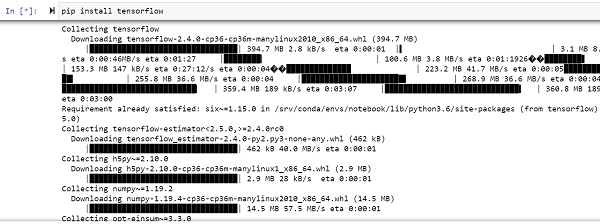# How can ‚Äòplaceholders‚Äô in Tensorflow be used while multiplying matrices?

Tensorflow is a machine learning framework that is provided by Google. It is an open−source framework used in conjunction with Python to implement algorithms, deep learning applications and much more. It is used in research and for production purposes.

It has optimization techniques that help in performing complicated mathematical operations quickly. This is because it uses NumPy and multi−dimensional arrays. These multi−dimensional arrays are also known as ‘tensors’. The framework supports working with deep neural network. It is highly scalable, and comes with many popular datasets.

The ‘tensorflow’ package can be installed on Windows using the below line of code −

pip install tensorflow

Tensor is a data structure used in TensorFlow. It helps connect edges in a flow diagram. This flow diagram is known as the ‘Data flow graph’. Tensors are nothing but multidimensional array or a list.

We will be using the Jupyter Notebook to run these codes. TensorFlow can be installed on Jupyter Notebook using ‘pip install tensorflow’.Following is an example −

## Example

import tensorflow.compat.v1 as tf
tf.disable_v2_behavior()
import numpy as np

mat_1 = tf.constant([[3, 5]])

a = tf.placeholder(tf.int32, shape=(2, 1))
b = tf.placeholder(tf.int32)

product = tf.matmul(mat_1, a) + b

with tf.Session() as sess:
result = sess.run(product, feed_dict={a: np.array([,]), b:1})
print(result)

result = sess.run(product, feed_dict={a: np.array([,]), b:3})
print(result)

## Output

[]
[]

## Explanation

• Import the required packages and provide an alias for it, for ease of use.

• The ‘tf.constant’ maps the input data as a constant value. These constants are a apart of the computation graph that runs with the input data.

• If a different input data is required, the ‘tf.constant’ can be replaced with ‘tf.placeholder’.

• The ‘feed_dict’ function is used to map different input data to placeholder.

• The product of the matrices is computed.

• This output is displayed on the console.